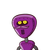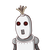# in how many years would RS 430000 become RS 497778.75 at 5% p.a compund interest..?​

in how many years would RS 430000 become RS 497778.75 at 5% p.a compund interest..?​

### 2 thoughts on “in how many years would RS 430000 become RS 497778.75 at 5% p.a compund interest..?​”

1.Almost 5 years. Sorry this is not my specialty.

2.3 years

Step-by-step explanation:

using formula;-

future value=R [1+i]^n

497778.75=430000[1+0.05]^n

1.157625=[1.05]^n

taking log on b/s

log(1.157625)=n log(1.05)

n=0.06357/0.02119